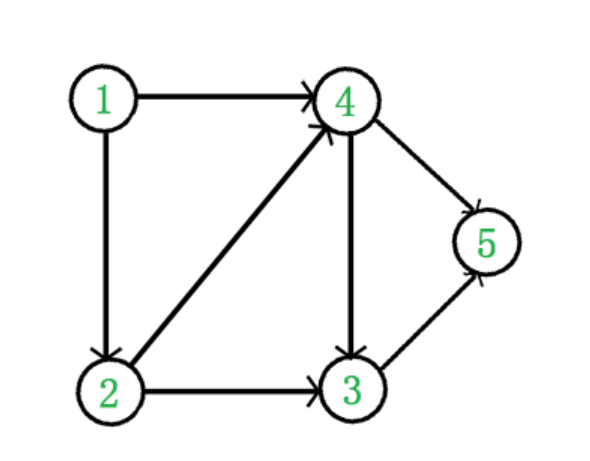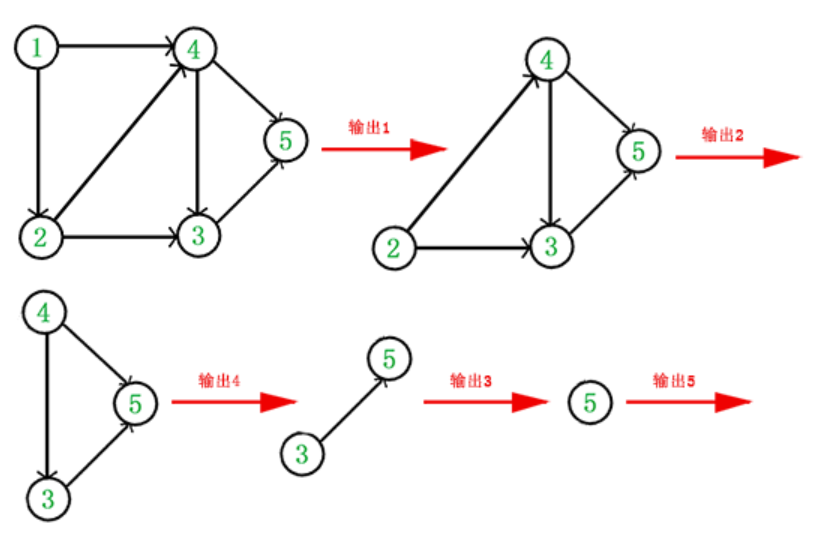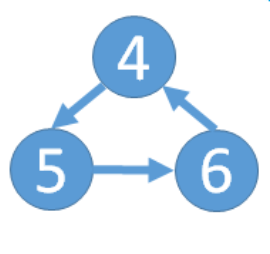# 拓扑排序-有向无环图（DAG, Directed Acyclic Graph）

1.每个顶点出现且只出现一次。

2.若存在一条从顶点 A 到顶点 B 的路径，那么在序列中顶点 A 出现在顶点 B 的前面。1.首先将边与边的关系确定，建立好入度表和邻接表。

2.从入度为0的点开始删除，如上图显然是1的入度为0，先删除。3.于是，得到拓扑排序后的结果是 { 1, 2, 4, 3, 5 }。通常，一个有向无环图可以有一个或多个拓扑排序序列。因为同一入度级别的点可以有不同的排序方式。

4.拓扑排序可以判断图中有无环，如下图#### 输出

Sample Input

2
2 2
1 2
2 1
3 2
1 2
1 3

Sample Output

Wrong
Correct
#include<stdio.h>
#include<algorithm>
#include<iostream>
#include<vector>
#include<stack>
#include<queue>
#include<cstring>
using namespace std;
const int maxa=100100;
vector<int>vec[maxa];
queue<int>q;
int rudu[maxa];
int t,n,m,x,y,now;
bool tuopu(){
int cnt=0;
while(!q.empty()) q.pop();
for(int i=1;i<=n;i++)
if(rudu[i]==0) q.push(i);
while(!q.empty()){
now=q.front();
cnt++;
q.pop();
for(size_t i=0;i<vec[now].size();i++)
if(--rudu[vec[now][i]]==0)
q.push(vec[now][i]);
}
if(cnt==n) return true;
else return false;
}

int main(){
cin>>t;
while(t--)
{
cin>>n>>m;
memset(rudu,0,sizeof(rudu));
for(int i=1;i<=n;i++) vec[i].clear();
while(m--)
{
cin>>x>>y;
vec[x].push_back(y);
rudu[y]++;//入度+1
}
if(tuopu()) cout<<"Correct"<<endl;
else cout<<"Wrong"<<endl;
}
return 0;
}

posted @ 2019-02-18 15:21  守林鸟  阅读(2178)  评论(0编辑  收藏